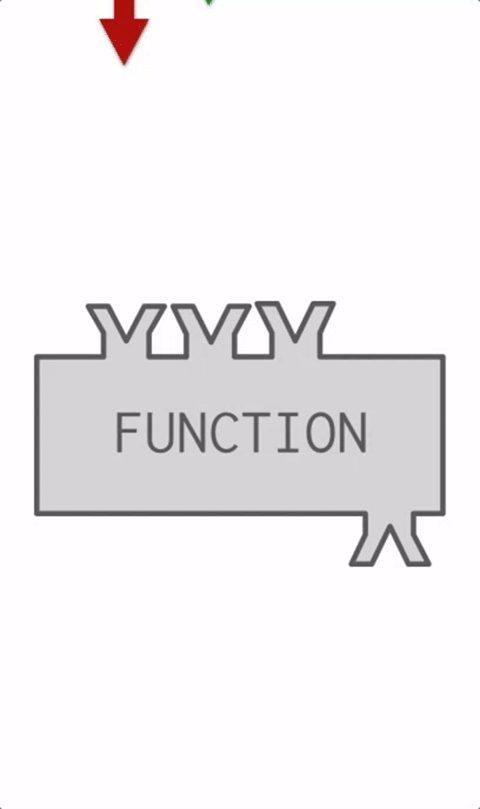# Chapter 2b - Built-in FunctionsAnimation## Built in Functions​

👀

### Using + with strings.​

we know that when we use `+` with numbers, the program will give us the mathematic operation of the sum of the numbers. But what happens when we have words?

``s1 = "Agent" # assign values to string 1 and string 2s2 = "007"print ("Using + with strings ")print("String 1: " + s1) # print values of s1 and s2print("String 2: " + s2)print ("String 1 + String 2: " + s1 + s2) # concatenation of strings - Line 18print("")``
🧪 Try the code out!

### str(), min(), max()​

``# Part 1a = 12 # assign value to variable ab = 3 # assign value to variable bc = 4 # assign value to variable cprint ("a: " + str(a) + " b: " + str(b) + " c: " + str(c) ) # print values for a, b and cprint ("Minimum of a, b and c is " + str(min(a,b,c) ) ) # print minimum value among a, b and c  # Line 8print ("Maximum of a, b and c is "+ str(max(a, b, c) ) ) # print maximum value among a, b and c  # Line 9print("") # print an empty lineprint("")``
🧪 Try the code out!

### int() Converting Words into Integers​

``# Part 3a = 12 # assign value to variable as2 = "007"print ("A string which holds a number can be changed to number data type like this:")s2_as_a_num = int(s2) # typecasting a number into a stringprint("sum of a and s2_as_a_num: " + str(s2_as_a_num + a)) # adding a typecasted number with another``
🧪 Try the code out!

### pow() and abs()​

👀

``a = 8b = 2c = -9d = 9.9print(pow(a,b)) # returns a raised to the power bprint(pow(d,0)) # returns d raised to the power of 0print(abs(c)) # returns the absolute value of cprint(abs(d)) # returns the absolute value of d``
🧪 Try the code out!

### Exercise​

👀

##### 📝Exercise: Counting Apples

write a program that tracks the mumber of apples the user ends up with after starting with a set number of apples. Students can set the initial number of apples to a number of their choice. The output should follow this script:

``You have ___ apples.You plant one tree from a seed you found in your attic and harvest five apples from it. You now have ___ apples.You extract seeds from every apple you have, discard the apples, and plant all the seeds. You harvest four times as many apples as you had. You now have ___ apples.You look over your apples and realize some of the trees were diseased! You have to throw out half of your apples. You now have ___ apples.You decide to bake an apple pie. This requires six apples. You now have ___ apples.``

Example Output

``You have 20 apples.You plant one tree from a seed you found in your attic and harvest five apples from it. You now have 25 apples.You extract seeds from every apple you have, discard the apples, and plant all the seeds. You harvest four times as many apples as you had. You now have 100 apples.You look over your apples and realize some of the trees were diseased! You have to throw out half of your apples. You now have 50.0 apples.You decide to bake an apple pie. This requires six apples. You now have 44.0 apples.``
✍ You can solve the problem here using Trinket